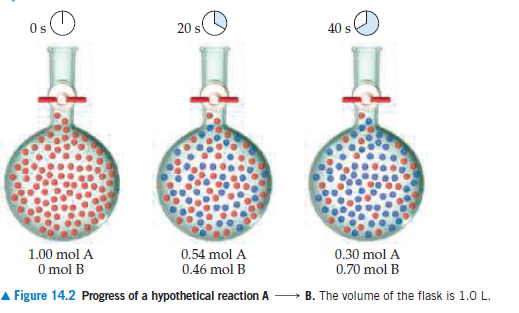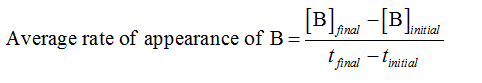# Os40 s1.00 mol A0.54 mol A0.46 mol B0.30 mol A0.70 mol BO mol BA Figure 14.2 Progress of a hypothetical reaction AB. The volume of the flask is 1.0 L.

Question
1 views

Use the data in Figure 14.2 to calculate the average rate of
appearance of B over the time interval from 0 s to 40 s.help_outlineImage TranscriptioncloseOs 40 s 1.00 mol A 0.54 mol A 0.46 mol B 0.30 mol A 0.70 mol B O mol B A Figure 14.2 Progress of a hypothetical reaction A B. The volume of the flask is 1.0 L. fullscreen
check_circle

Step 1

Rate of reaction represents the change of concentration of a reactant or a product with respect to time. It can be expressed either by reduce amount of reactant in per unit time or increase amount of product in per unit time.

Step 2

Given information:

• At t = 0, mole of B = 0
• At t = 20 s, mole of B = 0.46
• At t = 40 s, mole of B = 0.70
• Volume = 1.0 L
Step 3

The average rate of appearance of B over the time interval from 0 s to 40 s is calculated as follows:...

### Want to see the full answer?

See Solution

#### Want to see this answer and more?

Solutions are written by subject experts who are available 24/7. Questions are typically answered within 1 hour.*

See Solution
*Response times may vary by subject and question.
Tagged in